Refer to our Texas Go Math Grade 8 Answer Key Pdf to score good marks in the exams. Test yourself by practicing the problems from Texas Go Math Grade 8 Module 1 Quiz Answer Key.

1.1 Rational and Irrational Numbers

Write each fraction as a decimal.

Question 1.
$$\frac{7}{20}$$ ____
To write $$\frac{7}{20}$$ as a decimal. we divide the numerator by the denominator until the remainder is zero or until the digits in the quotient to repeat. We add as many zeros after the decimal point in the dividend as needed.$$\frac{7}{20}$$ = 0.35

Question 2.
$$\frac{14}{11}$$ ____
Let x = $$1 . \overline{27} .$$ Since $$1 . \overline{27}$$ has two repeating digits multiply both sides of the equation with 100;
x = $$1 . \overline{27} .$$
100x = 100 × $$1 . \overline{27} .$$
100x = $$127 . \overline{27}$$ Subtract x = $$1 . \overline{27}$$ from both sides
99x = 126
x = $$\frac{14}{11}$$ = 1$$\frac{3}{11}$$
x = 1$$\frac{3}{11}$$

Question 3.
1$$\frac{7}{8}$$ ____
First, we convert the mixed number to an improper fraction:
1$$\frac{7}{8}$$ = 1 + $$\frac{7}{8}$$
= $$\frac{8}{8}$$ + $$\frac{7}{8}$$
= $$\frac{15}{8}$$
To write $$\frac{15}{8}$$ as a decimal, we divide the numerator by the denominator until the remainder is zero or until the digits in the quotient begin to repeat.
We add as many zeros after the decimal point in the dividend as needed.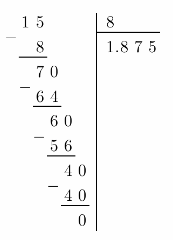1$$\frac{7}{8}$$ = 1.875

Find the two square roots of each number.

Question 4.
81 ____
x2 = 81
$$\sqrt{x^{2}}$$ = $$\sqrt{81}$$ Take square root from both sides of the equation
x = ± 9

Question 5.
1600 ____
x3 = 343
$$\sqrt{x^{3}}$$ = $$\sqrt{343}$$ Take square root from both sides of the equation
x = 7

Question 6.
$$\frac{1}{100}$$ ____
x2 = $$\frac{1}{100}$$ Take square root from both sides of the equation
$$\sqrt{x^{2}}$$ = $$\sqrt{\frac{1}{100}}$$ Solve for x
x = ±$$\frac{1}{10}$$

Question 7.
A square patio has an area of 200 square feet. How long is each side of the patio to the nearest 0.05? ____
The area of a square is found by multiplying the side of the square by itself
Therefore, to find the side of the square, we have to take the square root of the area
Lets denote with A the area of the patio and with s each side of the square. We have:
A = 200
A = s . s
s = $$\sqrt{A}$$ = $$\sqrt{200}$$
Following the steps as in “Explore Activity 1”on page 9, we can make an estimation for the irrationaL number:
196 < 200 < 225
$$\sqrt{196}$$ < $$\sqrt{200}$$ < $$\sqrt{225}$$
14 < $$\sqrt{200}$$ < 15
We see that 200 is much closer to 196 than to 225, therefore the square root of it should be between 14 and 14.5 To make a better estimation, we pick some numbers between 14 and 14.5 and calculate their squares:
14.12 = 198.81
14.22 = 201.64
14.1 < $$\sqrt{200}$$ < 14.2
$$\sqrt{200}$$ ≈ 14.15
We see that 200 is much closer to 14.1 than to 14.2, therefore the square root of it should be between 14.1 and 14.15.
If we round to the nearest 0.05, we have:
s = 14.15

Each side of the patio is 14.15 feet long.

1.2 Sets of Real Numbers

Write all names that apply to each number.

Question 8.
$$\frac{121}{\sqrt{121}}$$ _____
Using $$\sqrt{121}$$ = 11 we can see that $$\frac{121}{\sqrt{121}}$$ = $$\frac{121}{11}$$ = 11
Therefore it is whole, integer, rational, real

Whole, integer, rational, real

Question 9.
$$\frac{\pi}{2}$$ _____
π is an irrational. number. When multiplying any rational number (in this case $$\frac{1}{2}$$) with an irrational number we get an irrational number.
Therefore it is an irrational, real number.

Irrational, real.

Question 10.
Tell whether the statement “All integers are rational numbers” is true or false. Explain your choice.
All integers are rational numbers” is true, because every integer can be expressed as a fraction with denominator equal to 1. The set of integers is a subset of rational numbers.

True

1.3 Ordering Real Numbers

Compare. Write <>, or =.

Question 11.Given
$$\sqrt{8}$$ + 3 ? 8 + $$\sqrt{8}$$ Given

4 < 8 < 9 Approximate $$\sqrt{8}$$ Approximate $$\sqrt{8}$$
$$\sqrt{4}$$ < $$\sqrt{8}$$ < $$\sqrt{9}$$
2 < $$\sqrt{8}$$ < 3

1 < 3 < 4
$$\sqrt{1}$$ < $$\sqrt{3}$$ < $$\sqrt{4}$$
1 < $$\sqrt{3}$$ < 2

$$\sqrt{8}$$ + 3 is between 5 and 6. Use the estimations to simplify the expressions
8 + $$\sqrt{3}$$ is between 9 and 10.

$$\sqrt{8}$$ + 3 < 8 + $$\sqrt{3}$$ Compare

$$\sqrt{8}$$ + 3 < 8 + $$\sqrt{3}$$

Question 12.Approximate the value of $$\sqrt{5}$$ by comparing the number 5 to the closest perfect squares:
4 < 5 < 9
$$\sqrt{4}$$ < $$\sqrt{5}$$ < $$\sqrt{9}$$
2 < $$\sqrt{5}$$ < 3
So, $$\sqrt{5}$$ lies between 2 and 3.

In the same way approximate the value of $$\sqrt{11}$$ by comparing the number 11 to the closest perfect squares:
9 < 11 < 16
$$\sqrt{9}$$ < $$\sqrt{11}$$ < $$\sqrt{16}$$
3 < $$\sqrt{11}$$ < 4 So, $$\sqrt{11}$$ lies between 3 and 4. $$\sqrt{5}$$ + 11 lies between 13 and 14, while $$\sqrt{11}$$ + 5 lies between 8 and 9. Therefore: $$\sqrt{5}$$ + 11 > $$\sqrt{11}$$ + 5

Order the numbers from least to greatest.

Question 13.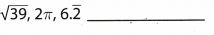Comparing 99 to the closest perfect squares gives us a estimation of $$\sqrt{99}$$:
81 < 99 < 100
$$\sqrt{81}$$ < $$\sqrt{99}$$ < $$\sqrt{10}$$
9 < $$\sqrt{99}$$ < 10
A good approximation for $$\sqrt{99}$$ is 9.95 because 9.952 ≈ 99
Second, π ≈ 3.14 ⇒ π2 ≈ (3.14)2 ≈ 9.86
Also, $$9 . \overline{8}$$ ≈ 9.88
So because of: 9.86 < 9.88 < 9.95 the order is π2, $$9 . \overline{8}$$, $$\sqrt{99}$$

Question 14.$$\sqrt{\frac{1}{25}}$$, $$\frac{1}{4}$$, $$0 . \overline{2}$$ Given
$$\sqrt{\frac{1}{25}}$$ = $$\frac{1}{5}$$ = 0.2 Evaluate
$$\frac{1}{4}$$ = 0.25 Evaluate
$$0 . \overline{2}$$ = 0.222 ≈ 0.22 Underlined number is the repeating number after decimal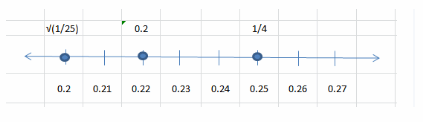Graph on the number line.
$$\sqrt{\frac{1}{25}}$$, $$0 . \overline{2}$$, $$\frac{1}{4}$$ From least to greatest.

Essential Question

Question 15.
How are real numbers used to describe real-world situations?
In real-word situations we use real numbers to count or make measurements. They can be seen as a convention for us to quantify things around, for example the distance, the temperature, the height etc.

Texas Go Math Grade 8 Module 1 Mixed Review Texas Test Prep Answer Key

Texas Test Prep

Selected Response

Question 1.
The square root of a number is 9. What is the other square root?
(A) -9
(B) -3
(C) 3
(D) 81
(A) -9

Explanation:
We know that every positive number has two square roots, one positive and one negative. We are given the
principal square root (9), so the other square root would be its negative (-9).
To prove that, we square both numbers and we compare the resuLts:
9 . 9 = 81
(-9) . (-9) = 81

Question 2.
A square acre of land is 4840 square yards. Between which two integers is the length of one side?
(A) between 24 and 25 yards
(B) between 69 and 70 yards
(C) between 242 and 243 yards
(D) between 695 and 696 yards
(B) between 69 and 70 yards

Explanation:
The area of a square is found by multiplying the side of the square by itself.
Therefore, to find the side of the square, we have to take the square root of the area.
Let’s denote with À the area of the Land and with s each side of the square. We have:
À = 4840
A = s . s
s = $$\sqrt{A}$$ = $$\sqrt{4840}$$
Following the steps as in “Explore Activity 1” on page 9, we can make an estimation for the irrational number: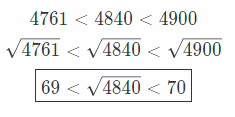Each side of the land is between 69 and 70 yards.

Question 3.
Which of the following is an integer but not a whole number?
(A) -9.6
(B) -4
(C) 0
(D) 3.7
(B) -4

Explanation:
-4 is an integer but not a whole number Whole numbers are not negative

Question 4.
Which statement is false?
(A) No integers are irrational numbers.
(B) All whole numbers are integers.
(C) No real numbers are irrational numbers.
(D) All integers greater than 0 are whole numbers.
(C) No real numbers are irrational numbers.

Explanation:
Option C is false Rational and irrational numbers are real numbers.

Question 5.
Which set of numbers best describes the displayed weights on a digital scale that shows each weight to the nearest half pound?
(A) whole numbers
(B) rational numbers
(C) real numbers
(D) integers
(B) rational numbers

Explanation:
Rational number The scale weigh nearest $$\frac{1}{2}$$ to pound

Question 6.
Which of the following is not true?(C)

Explanation:

A.
π ≈ 3.14 ⇒ π2 ≈ (3.14)2 ≈ 9.86
2π + 4 ≈ 10.28
It follows that π2 < 2π + 4 so the given statement is true B. π ≈ 3.14 ⇒ 3π ≈ 3 × 3.14 = 9.42 Number 9 is given It follows that 3π > 9 so the given statement is true

C.
$$\sqrt{27}$$ ≈ 5.2 because 5.22 = 27.04 ⇒ $$\sqrt{27}$$ + 3 ≈ 8.2
$$\frac{17}{2}$$ = 8$$\frac{1}{2}$$ = 8.5
It follows that $$\sqrt{27}$$ + 3 < $$\frac{17}{2}$$, so the given statement is not true

D.
$$\sqrt{24}$$ ≈ 4.9 because 4.92 = 24.01 ⇒ 5 – $$\sqrt{24}$$ ≈ 0.101
Number 1 is given.
It follows that 5 – $$\sqrt{24}$$ < 1 so the given statement is true

Option (C) is True

Question 7.
Which number is between $$\sqrt{21}$$ and $$\frac{3 \pi}{2}$$?
(A) $$\frac{14}{3}$$
(B) 2$$\sqrt{6}$$
(C) 5
(D) π + 1
(A) $$\frac{14}{3}$$

Explanation:
$$\sqrt{21}$$ and $$\frac{3 \pi}{2}$$ Given
$$\sqrt{21}$$ = 4.58 Evaluate
$$\frac{3 \pi}{2}$$ = $$\frac{3 * 3.14}{2}$$ = 4.71

$$\frac{14}{3}$$ = 4.67 Option A

2$$\sqrt{6}$$ = 4.90 Option B

5 Option C

π + 1 = 3.14 + 1 = 4.14 Option D

Question 8.
What number is shown on the graph?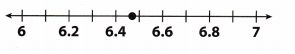(A) π + 3
(B) $$\sqrt{20}$$ + 2
(C) $$\sqrt{14}$$ + 2.5
(D) $$6 . \overline{14}$$
(B) $$\sqrt{20}$$ + 2

Explanation:
Approximate every option and see which fits the point showed on the graph.
We can see that the given point on the graph lies between 6.4 and 6.5 but closer to 6.5.
π + 3 ≈ 3.14 + 3 = 6.14 This option obviously isn’t our answer because it isn’t between 6.4 and 6.5.
Next
$$\sqrt{4}$$ + 2.5 = 2 + 2.5 = 4.5 This option also obviously isn’t our answer because it isn’t between 6.4 and 6.5
Next
$$\sqrt{20}$$ + 2 ≈ 4.47 + 2 = 6.47 We can see that this fits out graph since it is between 6.4 and 6.5
Finally,
$$6 . \overline{14}$$ ≈ 6.14 This option also obviously isn’t our answer because it isn’t between 6.4 and 6.5
So the final answer is that only the option C is shown on the graph.

Question 9.
Which list of numbers is in order from least to greatest?
(A) 3.34, $$\frac{10}{3}$$, π, $$\frac{11}{4}$$
(B) $$\frac{10}{3}$$, 3.3, $$\frac{11}{4}$$, π
(C) π, $$\frac{10}{3}$$, $$\frac{11}{4}$$, 3.3
(D) $$\frac{11}{4}$$, π, 3.3, $$\frac{10}{3}$$
(D) $$\frac{11}{4}$$, π, 3.3, $$\frac{10}{3}$$

Explanation:
• To write $$\frac{10}{3}$$ as a decimal, we divide the numerator by the denominator until the remainder is zero or until the digits in the quotient begin to repeat.
We add as nìanv zeros after the decimal point in the dividend as needed.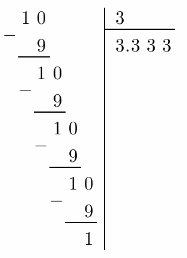when a decimal lias one or more digits that repeat indefinitely, we write the decimal with a bar over the repeating digit(s). In our case, 3 repeats indefinitely.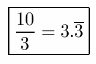• To write $$\frac{11}{4}$$ as a decimal, we divide the numerator by the denominator until the remainder is zero or until the digits in the quotient begin to repeat.
We add as many zeros after the decimal point in the dividend as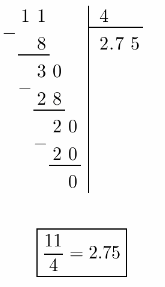Plot numbers on number line: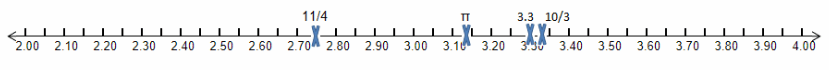Gridded Response

Question 10.
What is the decimal equivalent of the fraction $$\frac{28}{25}$$?a. To find the length of an edge (x) substitute V = 1728 and solve for X by taking cube roots from both sides of
the equation:
1728 = x3
x = 12
So the length of the edge is 12 inches.

b. Since cubes are made of squares, what we really need to calculate is the area of a square that has a length of
the side x = 12
Use formula for area of a square A = x2:
A = x2 = 122 = 144 inches.
So, the area of one side of the cube is 144 square inches.

c. Like we said in b., a cube is made out of squares, precisely, it is made out of 6 squares.
To find the surface area of the cube we need to add all the areas from the squares that make the cube, so:
S = 6 × r = 6 × 114 = 864 inches squared
So, the surface area of the cube is 864 square inches.

d. To get the desired surface area in square feet divide the surface area in square inches by 144 (since 1 ft = 12 in
⇒ 1 ft2 = 144 in2)
S = $$\frac{864}{144}$$ = 6 square feet.

a. x = 12 inches
b. A = 144 square inches
c. S = 864 square inches
d. S = 6 square feet

Scroll to Top